# JP4378120B2 - Operation control system for home cogeneration system - Google Patents

## Info

Publication number
JP4378120B2
JP4378120B2 JP2003192712A JP2003192712A JP4378120B2 JP 4378120 B2 JP4378120 B2 JP 4378120B2 JP 2003192712 A JP2003192712 A JP 2003192712A JP 2003192712 A JP2003192712 A JP 2003192712A JP 4378120 B2 JP4378120 B2 JP 4378120B2
Authority
JP
Japan
Prior art keywords
heat
power
amount
Prior art date
Legal status (The legal status is an assumption and is not a legal conclusion. Google has not performed a legal analysis and makes no representation as to the accuracy of the status listed.)
Active
Application number
JP2003192712A
Other languages
Japanese (ja)
Other versions
JP2005030211A (en
Inventor

Original Assignee

Priority date (The priority date is an assumption and is not a legal conclusion. Google has not performed a legal analysis and makes no representation as to the accuracy of the date listed.)
Filing date
Publication date
Application filed by 東邦瓦斯株式会社 filed Critical 東邦瓦斯株式会社
Priority to JP2003192712A priority Critical patent/JP4378120B2/en
Publication of JP2005030211A publication Critical patent/JP2005030211A/en
Application granted granted Critical
Publication of JP4378120B2 publication Critical patent/JP4378120B2/en
Application status is Active legal-status Critical
Anticipated expiration legal-status Critical

## Images

•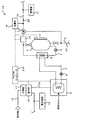•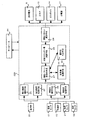•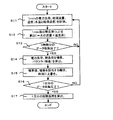•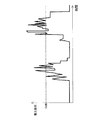•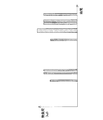•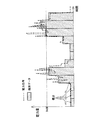•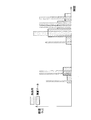•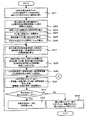•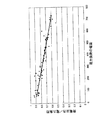•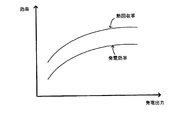•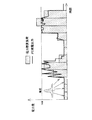•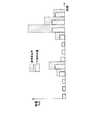•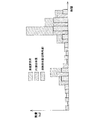•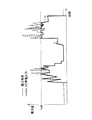•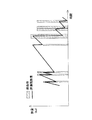•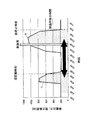•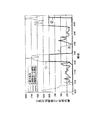••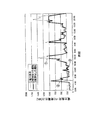••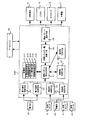•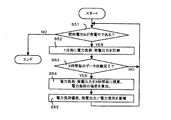••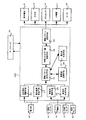•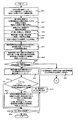•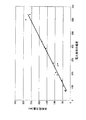•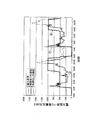•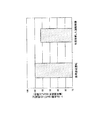•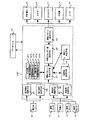## Classifications

• YGENERAL TAGGING OF NEW TECHNOLOGICAL DEVELOPMENTS; GENERAL TAGGING OF CROSS-SECTIONAL TECHNOLOGIES SPANNING OVER SEVERAL SECTIONS OF THE IPC; TECHNICAL SUBJECTS COVERED BY FORMER USPC CROSS-REFERENCE ART COLLECTIONS [XRACs] AND DIGESTS
• Y02TECHNOLOGIES OR APPLICATIONS FOR MITIGATION OR ADAPTATION AGAINST CLIMATE CHANGE
• Y02EREDUCTION OF GREENHOUSE GAS [GHG] EMISSIONS, RELATED TO ENERGY GENERATION, TRANSMISSION OR DISTRIBUTION
• Y02E20/00Combustion technologies with mitigation potential
• Y02E20/10Combined combustion
• Y02E20/14Combined heat and power generation [CHP]
• YGENERAL TAGGING OF NEW TECHNOLOGICAL DEVELOPMENTS; GENERAL TAGGING OF CROSS-SECTIONAL TECHNOLOGIES SPANNING OVER SEVERAL SECTIONS OF THE IPC; TECHNICAL SUBJECTS COVERED BY FORMER USPC CROSS-REFERENCE ART COLLECTIONS [XRACs] AND DIGESTS
• Y02TECHNOLOGIES OR APPLICATIONS FOR MITIGATION OR ADAPTATION AGAINST CLIMATE CHANGE
• Y02TCLIMATE CHANGE MITIGATION TECHNOLOGIES RELATED TO TRANSPORTATION
• Y02T10/00Road transport of goods or passengers
• Y02T10/10Internal combustion engine [ICE] based vehicles
• Y02T10/16Energy recuperation from low temperature heat sources of the ICE to produce additional power
• Y02T10/166Waste heat recovering cycles or thermoelectric systems

## Description


BACKGROUND OF THE INVENTION
The present invention relates to an operation control system for a home cogeneration system that includes a cogeneration device that generates electric power and heat and controls the operation of the home cogeneration system configured to obtain both electric power and heat.

[Prior art]
Household cogeneration systems are installed in each home, supplying generated power, and recovering waste heat to cover hot water and heating applications. Is expected.

As an operation control system of such a home cogeneration system, for example, there is one disclosed in Patent Document 1. According to the operation control system of this home cogeneration system, the power load pattern and the heat load pattern over time are stored as a power load pattern and a heat load pattern for a predetermined period of time such as one day. Energy efficiency when operating a combined heat and power unit such as a gas engine based on the heat load pattern. The power load is covered by the power generation output during the high energy saving time, and the power is output during the low energy saving time. Since the start time and stop time of the cogeneration device are determined so as to cover the load with commercial power, and an operation pattern is created, it is possible to increase power generation efficiency, suppress generation of surplus power, and improve power generation efficiency .

[Patent Document 1]
JP 2002-213303 A (see paragraphs 0055 to 0074, FIGS. 8 and 9).

[Problems to be solved by the invention]
However, the operation control system of the conventional home cogeneration system uses a plurality of calculation formulas (in the embodiment, Formulas 51 to 51) using the power load data and the heat load data for one section (30 minutes in the embodiment). 68, Formulas 45 to 50), the primary energy conversion values in the operation state and the operation stop state of the section are calculated, respectively. In order to calculate the primary energy for one day, the number of calculations is It was considerably large (in the example, 2 to the 48th power). Then, a large number of primary energy conversion values (in the example, 2 to the 48th power) obtained by the calculation formula are input to the comparison means, and are sequentially compared, leaving a small primary energy conversion value, and finally the primary energy conversion value. The combination of the operation state where the energy is minimum and the operation stop state is obtained as the optimum operation state. Therefore, the operation control system of the conventional home cogeneration system has a lot of data to be handled and the number of calculations, and it has been difficult to use it with an actual control device. Also, when using a household cogeneration system that can follow the power load, such as a fuel cell, for an average processed power load data for one section, for example, 30 minutes, how many Wh can be generated, how many Wh? It was not realistic because there was no important logic for heat recovery.

Therefore, the present invention has been made to solve the above-described problem, and can be used for home use that can realize operation control that exhibits more energy savings based on a relatively small amount of data by a simple determination logic. It aims at providing the operation control system of a cogeneration system.

[Means for Solving the Problems]
In order to solve the above problems, the present invention has the following configuration.

(2) In the invention described in (1), the operation pattern provisional deciding means includes a measuring unit that measures the power generation output and the power load at a predetermined interval, and each time a predetermined time elapses between the power generation output and the power load measured by the measurement unit. And a measurement result storage unit that calculates a power load deviation and accumulates a value obtained by dividing the power generation output by the power load in association with the power load deviation, and the measurement result storage unit stores a predetermined number of data. Sometimes it is necessary to store new data after the correlation function calculation unit that calculates the correlation function between the power output divided by the power load and the power load deviation, and the measurement result storage unit stores a predetermined number of data Data rewrite unit that rewrites the oldest data stored in the measurement result storage unit to new data, and the data rewrite unit recalculates the correlation function when the data is rewritten a predetermined number of times. It has a correlation function correcting unit for correcting a, and sets the power output of the cogeneration unit using a correlation function that is modified by the correlation function correcting unit.

(3) In the invention described in (2), the operation pattern provisional deciding means has a correlation function between a value obtained by dividing the power generation output by the power load and a power load deviation for each season, and the correlation function corresponding to the season Is used to set the power generation output of the cogeneration device.

(4) In the invention according to any one of (1) to (3), heat calculated by calculating a heat dissipation loss that the heat storage device releases by the time of use of heat, and obtained based on the power generation output of the combined heat and power supply device It is characterized by having heat dissipation loss processing means for subtracting the heat dissipation loss from the recovered amount to calculate the actual heat recovery amount and determining the start time and stop time of the combined heat and power supply device.

(5) In the invention according to any one of (1) to (3), in order to store a predetermined amount of heat in the heat storage device, the combined heat and power supply device stores heat before the temporary stop time and temporary start time To determine the amount of energy consumed when heat is stored after the temporary start-up time, taking into consideration heat dissipation loss, and comparing the calculated energy consumption to select an operation pattern with a small amount of energy consumption It is characterized by having.


(7) In the invention described in (6), the operation pattern provisional deciding means integrates a measuring unit that measures the surplus power amount at a constant interval and the surplus power amount measured by the measuring unit every time a predetermined time elapses. , Calculating a power load deviation, and further storing a surplus power amount in association with the power load deviation, and a surplus power amount and a power load deviation when the measurement result storage unit accumulates a predetermined number of data. The correlation function calculation unit that calculates the correlation function between the measurement result and the measurement result accumulation unit accumulates a predetermined number of data, and when new data needs to be accumulated, A data rewriting unit that rewrites old data with new data, and a correlation function correction unit that recalculates and corrects the correlation function when the data rewriting unit rewrites the data a predetermined number of times. And sets the power output of the cogeneration unit using a correlation function which is corrected me.

(8) In the invention described in (7), the operation pattern provisional determination unit has a correlation function between the surplus power amount and the power load deviation for each season, and uses the correlation function according to the season, The power generation output is set.

(9) In the invention according to any one of (6) to (8), the heat obtained by the heat storage device before the use time of heat is calculated, and the heat obtained based on the power generation output of the combined heat and power supply device It is characterized by having heat dissipation loss processing means for subtracting the heat dissipation loss from the recovered amount to calculate the actual heat recovery amount and determining the start time and stop time of the combined heat and power supply device.

(10) In the invention according to any one of (6) to (8), in order for the heat and power supply device to store a predetermined amount of heat in the heat storage device, the temporary stop time is changed and before the temporary stop time. Calculate the amount of energy consumed when storing heat and when changing the temporary start time and storing heat after the temporary start time in consideration of the heat dissipation loss, and compare the calculated amount of energy consumed to reduce the amount of energy consumed. It has the advantage judgment means to choose.

Then, the effect | action of the invention which has the said structure is demonstrated.

Next, the integrated value of the power load and the power load deviation corresponding to a predetermined condition (for example, a specific day of the week) are read, and the combined heat and power supply is operated following the power load so as to cover the total heat load of the day. The power generation output, power generation efficiency, heat recovery rate, power purchase amount, and heat recovery amount are determined, and the start time and stop time of the combined heat and power supply device at this time are provisionally determined as the operation pattern.
On the other hand, when the home cogeneration system is equipped with a thermoelectric conversion device, the surplus power of the cogeneration device can be converted into heat by the thermoelectric conversion device and used, so that the total heat load of the day can be covered. Obtain the power generation output, power generation efficiency, power purchase amount, heat recovery amount, and conversion heat amount when the cogeneration device is operated to output in stages according to the power load, and the start time and stop time of the cogeneration device at this time Is temporarily determined as a driving pattern.

Next, the amount of energy consumed is calculated by obtaining the amount of fuel consumed, the amount of electricity purchased, and the like consumed when the cogeneration apparatus is operated based on the temporarily determined operation pattern.
In this way, the amount of energy consumption is calculated while sequentially shifting the start time, the calculated amount of energy consumed is compared, the operation pattern having the start time and the stop time that minimizes the amount of energy consumption is selected, and combined heat and power Operate the device.

As described above, the present invention reduces the amount of data by using the deviation between the power load and the thermal load, and when the home cogeneration system uses the deviation to perform load following or stepwise output, for example, one section, for example, The power generation output amount and the heat recovery amount with high accuracy can be determined for the power load data every 30 minutes or 1 hour. In addition, the startup time is shifted sequentially to calculate the amount of energy consumed for the start / stop times that are provisionally determined to cover the amount of heat required for the day or most of it, and the operation pattern that minimizes the amount of energy consumption is selected. Since the combined heat and power unit is operated with high efficiency, after calculating and storing the amount of energy consumed in the operation status and the stop status every predetermined time, the operation pattern is created by comparing them. Compared to the system, it is possible to reduce the amount of data used when selecting the operation pattern based on the number of times of calculating the amount of energy consumption and the amount of energy consumption. Therefore, according to the present invention, it is possible to realize operation control that exhibits more energy savings based on a relatively small amount of data with a simple determination logic.


In addition, when the cogeneration apparatus is operated in a stepwise output operation, the surplus power amount can be predicted by the same method. Therefore, even in the case of a home cogeneration system including a thermoelectric conversion device, it is possible to set a highly accurate power generation output according to the household power load, the performance of the cogeneration device, and the like without complicated calculations.

In addition, the electric power equipment used at home changes every season, and the electric load also fluctuates with it. For this reason, for example, at the turn of the season, the power equipment to be used is not constant, and it is difficult to predict the power load and set the power generation output. Therefore, a correlation function is provided for each season, and when it is difficult to predict the power load or surplus power, such as at the turn of the season, the power generation output is set using the correlation function according to the season, and the operation pattern is determined. By doing so, it is possible to perform operation control that accurately follows the household power load regardless of disturbance factors such as the season.

By the way, since the heat stored in the heat storage device is generally dissipated as time elapses, the amount of heat recovered with the power generation of the combined heat and power supply device and the amount of heat stored in the heat storage device and used for actual use Does not necessarily match. Therefore, the heat dissipation loss rate is calculated from the outside air temperature and water temperature separately measured, and the time until the recovered heat is used is obtained from the heat recovery amount by the combined heat and power supply device. Obtain the actual heat recovery amount by subtracting the heat release amount from the heat recovery amount, and determine the start and stop times of the combined heat and power system to cover the total heat load of the day based on the actual heat recovery amount By doing so, more realistic operation control can be performed with high accuracy.

Further, the heat dissipation loss increases as the stop time of the combined heat and power unit becomes longer, for example, when the heat storage of the heat storage device is used in the morning of the next day after the combined heat and power supply unit is stopped at the end of the day. At this time, heat is collected before the temporary stop time on the predicted date to cover part or all of the heat load on the next day, and heat is supplied so as to cover only the heat load required on the predicted date before the temporary start time on the predicted date. It compares with the case where it collect | recovers and heat is not left on the next day, and judges whether it is efficient to collect heat | fever, and selects an operation pattern. That is, when the heat load of the next day is stored in the heat storage device before the temporary stop time for stopping the combined heat and power supply on the predicted date, and the heat load of the current day before the temporary start time for starting the combined heat and power supply device on the predicted date The amount of energy consumed when storing heat is calculated in consideration of heat dissipation loss, and an operation pattern having a start time and a stop time with a small amount of energy consumption is selected. In this way, since the start time and stop time are partially recalculated for the time zone with large heat dissipation loss without recalculating the entire operation pattern of the combined heat and power unit, a highly energy efficient operation pattern can be created with a small number of calculations. can do.

DETAILED DESCRIPTION OF THE INVENTION
(First embodiment)
Next, a first embodiment of the operation control system for a home cogeneration system according to the present invention will be described with reference to the drawings. FIG. 1 is a schematic configuration diagram of a home cogeneration system 1A.
The home cogeneration system 1A supplies electric power generated by a fuel cell (corresponding to a “heat and power supply device”) 8 to an electric power device 19 and also stores water heated by heat generated by power generation in a hot water storage tank. (Equivalent to “heat storage device”) 2 is stored in 2 and supplied to the thermal equipment 11.

The hot water storage tank 2 has a capacity of 100 to 200 L, and is always filled with water supplied from a water pipe 3 connected to the bottom. A circulation pipe 4 is connected to the bottom and top of the hot water storage tank 2, and the first pump 5 installed in the circulation pipe 4 is driven to take out the water in the hot water storage tank 2 from the bottom and return it to the top. ing. The circulation pipe 4 is provided with a heat exchanger 6 on the downstream side of the first pump 5, and is connected to the heat recovery circulation pipe 7 via the heat exchanger 6. The heat recovery circulation pipe 7 is connected to the fuel cell 8 having a maximum output of 1 kW, and the circulating water circulating through the heat recovery circulation pipe 7 recovers the exhaust heat of the fuel cell 8 by driving the second pump 9. It is like that. Therefore, if the first pump 5 and the second pump 9 are driven while the fuel cell 8 is generating electricity, the circulating water in the heat recovery circulation pipe 7 is heated by the exhaust heat of the fuel cell 8, and the heat exchanger 6, heat can be transferred from the circulating water in the heat recovery circulation pipe 7 to the water in the circulation pipe 4 to store hot water in the hot water storage tank 2.

An output circulation pipe 10 is connected to the upper part of the hot water storage tank 2 so as to supply hot water or heating hot water to a heating device 11 such as a kitchen or bath faucet or floor heating. The hot water supply temperature is detected by a temperature sensor 33 installed on the output circulation pipe 10. When the hot water supply temperature is higher than the set temperature, normal temperature tap water is added by the three-way valve 13, and the hot water supply temperature is set. When the temperature is lower than the temperature, the hot water is heated with a gas boiler (corresponding to an “auxiliary heating device”) 14. The amount of hot water stored in the hot water storage tank 2 is detected by sensing the boundary surface of the hot water and tap water with the temperature sensor 12, and the temperature of the tap water is detected by the temperature sensor 21 attached to the water pipe 3. In addition, the amount of hot water used is detected by a flow meter 15 installed downstream of the three-way valve 13.

The fuel cell 8 is connected to a power line 16 for taking out a power generation output, and is connected to a distribution board (corresponding to a “power purchase device”) 17. The distribution board 17 is also connected to a commercial power line 18 for supplying commercial power, and the power generation output and the commercial power are connected to the power equipment 19 such as a lighting fixture, a television, an air conditioner, and a personal computer by connecting the power generation output and the commercial power. It comes to supply. A power meter 20 is installed on the distribution board 17 to detect the amount of power consumed by the power device 19.
The fuel cell 8, the temperature sensor 12, the three-way valve 13, the gas boiler 14, the flow meter 15, the distribution board 17, the wattmeter 20, the temperature sensor 21, the temperature sensor 33, etc. include a microcomputer (corresponding to a “control device”) .) 22A is connected, and the operation of the fuel cell 8 is controlled by executing an operation control program stored in the microcomputer 22A.

FIG. 2 is a block diagram of the microcomputer 22A.
The microcomputer 22A has a learning function. The microcomputer 22A is connected to the database 34, and also includes a power load integrating means 23, a power load processing means 24, a heat load integrating means 25, a heat load processing means 26, an operation pattern provisional determining means 27, an energy consumption amount calculating means 28, An operation pattern selection unit 29, a heat dissipation loss processing unit 30, a superiority determination unit 31, and the like are provided.

The database 34 stores data such as electric power integration load, thermal load integration value, electric power load deviation, and thermal load deviation under certain conditions (for example, every week, every day of the week, every season, etc.).

The power load integrating means 23 samples the power load used by the power equipment 19 at a constant time interval by the power meter 20, integrates the sampled values, and overwrites the database 34.
The power load processing means 24 calculates a deviation for every predetermined time with respect to the sampling value of the power load sampled by the power meter 20 and stores the existing deviation stored in the database (corresponding to “power load deviation database”) 34. The power load deviation is rewritten.

The thermal load integrating means 25 samples the hot water supply temperature and the hot water supply flow rate at regular time intervals by the temperature sensor 12 and the flow meter 15, calculates the heat load consumed by the thermal device 11 from the hot water supply temperature and the hot water supply flow rate, and calculates the thermal load. It accumulates and overwrites the database 34.
The thermal load processing means 26 calculates a deviation for each predetermined time with respect to the thermal load calculated from the hot water supply temperature and the hot water supply flow rate, and stores the existing thermal load stored in the database (corresponding to “thermal load deviation database”) 34. The deviation is rewritten.

The operation pattern provisional decision means 27 reads the power load and the power load deviation based on a predetermined condition, and the power generation output when the fuel cell 8 performs the load following operation based on the read power load and the power load deviation, the power generation efficiency, The heat recovery rate, the amount of electricity purchased, and the amount of gas consumed when the gas boiler 14 is replenished are calculated, and the start time and stop time of the fuel cell 8 are provisionally determined. The operation pattern provisional decision means 27 has a unique correlation function according to the performance of the fuel cell 8 and the like, and shows the relationship between the power generation output and the power generation efficiency / heat recovery rate in order to determine the power generation output of the fuel cell 8. It has a relational expression.

Based on the operation pattern provisionally determined by the operation pattern provisional determination means 27, the energy consumption amount calculation means 28 is consumed by the gas boiler 14 when the amount of gas consumed, the amount of electricity purchased, and the hot water supply is insufficient. The amount of consumed energy is calculated by multiplying the amount of gas to be converted by a conversion constant necessary for calculating the primary energy amount.

The operation pattern selection means 29 compares the energy consumption amounts calculated by the energy consumption amount calculation means 28, and selects an operation pattern that minimizes the energy consumption amount.

The heat dissipation loss processing means 30 calculates the heat dissipation loss that the hot water storage tank 2 releases by the time of heat use, and subtracts the heat dissipation loss from the heat recovery amount obtained based on the power generation output of the fuel cell 8 to obtain the actual heat recovery amount. And the start time and stop time of the fuel cell 8 are determined so as to cover the heat load necessary for one day based on the actual heat recovery amount.

The superiority judging means 31 is configured to store the heat before the temporary stop time in order to store a predetermined amount of heat in the hot water storage tank 2 when the heat amount is predicted to be insufficient for the next heat load. Calculates the amount of energy consumed when changing the start-up time and storing heat after the temporary start-up time in consideration of heat dissipation loss, and compares the calculated amount of energy consumed to select an operation pattern with a small amount of energy consumption. is there.

Next, the operation of the operation control system of the home cogeneration system having the above configuration will be described with reference to a flowchart. The operation control system for household cogeneration systems mainly formulates power load prediction and heat load prediction based on the process of accumulating power load data and heat load data, and power load data and heat load data. The process of determining the operation pattern of the fuel cell 8 is performed in parallel.

FIG. 3 is a flowchart of the load data accumulation process. FIG. 4 is a diagram illustrating an example of power load data. FIG. 5 is a diagram illustrating an example of heat load data. FIG. 6 is a diagram illustrating the relationship between the power integration load and the power load over time. FIG. 7 is a diagram showing the relationship between the heat load and the heat integration load over time.

In the load data accumulation process, first, in S11, a one-minute power load, hot water flow rate, and temperature (water temperature and hot water temperature) are measured. That is, the power load is measured over time by the wattmeter 20, for example, as shown in FIG. The hot water supply flow rate is measured over time by the flow meter 15. Further, the temperature of the tap water is measured by the temperature sensor 21, and the hot water supply temperature is measured by the temperature sensor 33.

Next, in S12, a heat load (kJ) per minute is calculated (= flow rate of water × temperature difference). That is, the temperature load is calculated by subtracting the water temperature of the tap water from the hot water supply temperature, and the hot water flow rate is applied to the temperature difference to calculate the heat load (kJ) consumed by the thermal equipment 11 (see FIG. 5). At the same time, the hot water storage temperature is measured from the temperature sensor 12 installed in the hot water storage tank 2 of the fuel cell 8 to calculate the heat storage amount.
Next, in S13, it is determined whether or not data collection for one hour has been completed. If it is determined that data collection for one hour has not been completed (S13: NO), the process returns to S11 and data collection is continued. On the other hand, if it is determined that the data collection for one hour has been completed (S13: YES), the power load and the heat load are integrated in S14, and the variation (deviation) is calculated. In the present embodiment, the integrated value and the deviation are calculated at an interval of 1 hour, but can be arbitrarily set in view of the data storage capacity of the microcomputer 22A, for example, set in the range of about 15 minutes to 2 hours. May be.


Next, in S16, it is determined whether or not data collection for one day has been completed. If it is determined that the data collection for one day has not been completed (S16: NO), the process returns to S1 and the data collection is continued. On the other hand, when it is determined that the data collection for one day is completed (S16: YES), the total heat load for one day is calculated in S17. That is, the integrated heat load calculated at one hour intervals is further integrated to calculate the heat load consumed by the thermal device 11 in one day and stored in the database 34.

Next, power load / thermal load prediction and start / stop time determination processing will be described. FIG. 8 is a flowchart of power load / thermal load prediction and start / stop time determination processing. FIG. 11 is a diagram illustrating the power integration load and the power generation output over time. FIG. 9 is a diagram illustrating a correlation function between a value obtained by dividing the power generation output by the power load and the deviation. FIG. 10 is a diagram illustrating a relationship between power generation efficiency and power generation output. FIG. 12 is a diagram showing the relationship between the heat accumulation load and the heat recovery amount over time. FIG. 13 is a diagram showing the relationship between the accumulated heat load, the heat recovery amount, and the actual heat recovery amount over time. FIG. 14 is a graph showing the relationship between the power load and the power generation output when the fuel cell is operated according to the selected operation pattern over time. FIG. 15 is a diagram showing the relationship between the heat load and the amount of residual hot water stored when the fuel cell is operated according to the selected operation pattern.


Next, in S22, the power generation output when the load following operation is performed for two days is calculated from the power load and the deviation. At the same time, data necessary for calculating the amount of energy consumption is obtained. That is, in S23, for example, when the power load deviation read from the database 34 is 280, the value obtained by dividing the power generation output by the power load is determined from the correlation function shown in FIG. The power generation output as shown in FIG. 11 is obtained by multiplying the power integration load read from the power generation output by a value obtained by dividing the power generation output by the power load (here, 0.8). The power generation output is applied to the relationship diagram shown in FIG. 10, and the power generation efficiency and the heat recovery rate are obtained. Further, in S24, the amount of gas used as fuel for the home cogeneration system 1A is calculated by dividing the power generation output by the power generation efficiency. In S25, the heat recovery amount is calculated by multiplying the gas amount and the heat recovery rate. Furthermore, in S26, the heat dissipation rate per hour is calculated from the air temperature, water temperature, and the like.

Next, in S27, the stop time of the day before the predicted date is provisionally determined as the start time B1, and the remaining hot water amount at the start time B1 is calculated in consideration of the heat dissipation loss. That is, the amount of heat stored in the hot water storage tank 2 before starting the fuel cell 8 on the predicted date is calculated. In order to determine the power generation output time so as to cover the heat load necessary for one day, there is a limit to the amount of hot water stored in the hot water storage tank 2 (in this embodiment, 100 to 200 L), and hot water storage that can store heat on the predicted day. This is because it is necessary to grasp the amount.

Next, in S28, the amount of heat that can be recovered from the start time B1 is sequentially added. At that time, the heat recovery amount is taken into consideration the heat dissipation loss of the time difference between the recovery time and the use time. That is, for example, when the fuel cell 8 stops at 24:00 on the day before the predicted date, the 0:00 of the predicted date is provisionally determined as the activation time B1, and the amount of heat generated by the fuel cell 8 due to power generation is integrated from the activation time B1. Thus, the heat recovery amount is obtained (see FIG. 12). At this time, since the hot water storage tank 2 generates a heat dissipation loss until the hot water is used, the heat storage tank 2 is actually stored and used by subtracting the heat dissipation loss from the heat recovery amount. Therefore, the amount of heat obtained by subtracting the heat dissipation loss from the amount of heat recovered is integrated over time to obtain the actual amount of heat recovered (see FIG. 13). Further, the amount of heat required for the gas boiler 14 is also calculated. Considering these, the amount of heat that can be recovered in each time zone is added in order.

Next, in S29, the time when the total heat load of the day becomes smaller than the sum of the actual heat recovery amount and the actual hot water storage residual heat amount is temporarily determined as the stop time C1. That is, on the predicted date, the actual heat recovery amount taking into consideration the heat dissipation loss until the fuel cell 8 is recovered after being operated and used, and remains in the hot water storage tank 2 before starting the fuel cell 8 on the predicted date. In addition, if the remaining amount of heat stored in the hot water in consideration of the heat dissipation loss before use is stored in the hot water storage tank 2, and the heat storage covers the heat load on the predicted date, the fuel cell 8 can be operated efficiently. Is possible. Therefore, the time at which the total heat load on the predicted date can be covered by the actual heat recovery amount and the actual hot water storage residual heat amount is provisionally determined as the stop time C1 of the fuel cell 8.

Next, in S30, the daily energy consumption T1 is calculated and accumulated by adding the product obtained by multiplying the power purchase amount by the conversion factor and the product obtained by multiplying the gas amount by the conversion factor. At this time, the power purchase amount is calculated by subtracting the total power output from the total power load. That is, the energy saving performance of the home cogeneration system 1A can be determined based on the primary energy amount of the amount of power and gas consumed by the entire system including the fuel cell 8 and the gas boiler 14 in addition to the thermal device 11 and the power device 19. is there. Therefore, the amount of energy consumption required for the power load is calculated by subtracting the power generation output generated by the operation pattern from the total power load obtained by integrating the power load on the forecast date, and multiplying the power purchase amount by a conversion factor. Further, the amount of energy consumed for the household cogeneration system 1A and the heat load is obtained by multiplying the amount of city gas by a conversion factor. Then, by adding these energy consumption amounts, the energy consumption amount T1 of the operation pattern is calculated and stored in association with the operation pattern.

Next, in S31, it is determined whether or not the stop time C1 is equal to or less than 24: 00 + α of the next day. The reason that “24 o'clock + α on the next day” is used as a criterion is that a time zone with a high power load may occur across days. If it is determined that the stop time C1 is equal to or less than 24: 00 + α of the next day (S31: YES), the process proceeds to S32, and the activation time is delayed by 1 hour from Bi + 1 o'clock. Thereafter, the processing after S27 is performed, the stop time is provisionally determined as Ci + 1, and the energy consumption amount Ti + 1 is further accumulated.
In this manner, the start time B is shifted and fixed by one hour, and the operation pattern is sequentially created by temporarily determining the stop time C so that the operation is performed for the time required to recover the required amount of heat. Accumulate energy consumption.

If it is determined that the stop time C1 is not equal to or less than 24: 00 + α of the next day (S31: NO), the process proceeds to S33, where the minimum energy consumption Tmin, the start time Bmin, and the stop time Cmin are calculated. That is, the accumulated operation pattern is selected that minimizes the amount of energy consumed from start to stop (see FIG. 14). At this time, the same amount of energy consumption is stored as the tentatively determined operation pattern, and a large amount of energy consumption between the operation state and the stop state is obtained every predetermined time period (for example, 30 minutes) (the above-described conventional technology). Then, after storing them, it is possible to select an operation pattern with a smaller amount of data and the number of calculations than those in which the operation patterns are determined by comparing them (see the column of the prior art).

On the predicted date, the fuel cell 8 is operated according to the selected operation pattern (see FIG. 14), supplies hot water stored in the hot water storage tank 2 to the thermal equipment 11 (see FIG. 15), and generates power output. It supplies to the electric power apparatus 19 (refer FIG. 14). The power load that cannot be handled by the power generation output is covered by commercial power, and hot water that cannot be handled by the heat load is covered by heating with the gas boiler 14. When the hot water supply temperature is higher than a set temperature (for example, 42 ° C.), tap water is mixed with hot water at the three-way valve 13 and supplied to the thermal device 11.

FIG. 16 is a diagram illustrating an example of calculation logic related to heat dissipation loss.
For example, as shown in FIG. 16, when the peak of the thermal load is shown at 20:00, the hot water storage tank 2 is placed between 20:00 which shows the peak of the thermal load and 2:00 am which is the temporary stop time (X portion in the figure). When it is determined that the amount of heat stored can be stored in the hot water storage tank 2 by starting the fuel cell 8 before 10 o'clock (Y portion in the figure), which is the temporary start time, In which case, it is determined whether heat recovery is superior.

That is, after calculating the amount of heat collected in the hot water storage tank 2 from 20:00 indicating the peak of the heat load to the temporary stop time 2 (X portion in FIG. 16), the hot water in the hot water storage tank 2 is then used. The time is specified, and the heat dissipation loss radiated by the hot water storage tank 2 by the usage time is calculated based on the heat dissipation rate determined from the season and the outside temperature. Then, the amount of heat that can be used in the next heat load is calculated from the amount of heat recovered in the hot water storage tank 2 and the heat dissipation loss, and the actual heat efficiency is calculated based on the amount of city gas consumed by the operation of the fuel cell 8. Ask. Then, the total efficiency is calculated and stored from the actual heat efficiency and the power generation efficiency. The total efficiency may be calculated as an energy consumption amount.

Subsequently, the same amount of heat as the amount of heat stored in the hot water storage tank 2 from 20:00, which is the peak time of the heat load, to the temporary stop time 2:00 is collected before the temporary start time 10 o'clock (Y portion in FIG. 16). Consider the case. That is, the time necessary for recovering the same amount of heat as the heat stored in the hot water storage tank 2 from 20:00, which is the peak time of the thermal load, to 2:00, the temporary stop time, with reference to the temporary activation time of 10:00 is advanced. Set with. Here, for example, it is assumed that the fuel cell 8 is operated from 2 o'clock. In this case, the heat dissipation loss of the hot water storage tank 2 occurring from 2 o'clock to 10 o'clock provisional activation time is calculated based on the heat dissipation rate determined from the season, the outside air temperature and the like. Then, the amount of heat that can be used for the heat load of the next day is calculated from the amount of heat recovered in the hot water storage tank 2 and the heat dissipation loss, and is calculated based on the amount of city gas consumed by the operation of the fuel cell 8. Find thermal efficiency. Then, the total efficiency is calculated and stored from the actual heat efficiency and the power generation efficiency. The total efficiency may be calculated as an energy consumption amount.

Then, when the fuel cell 8 is operated to store heat from the peak time of the thermal load to the temporary stop time (X portion in FIG. 16), and when the fuel cell 8 is operated to store heat before the temporary start time (FIG. 16). Comparing the total efficiency with the Y portion), it is determined which one is superior in terms of the amount of energy consumption, the start time and the stop time of the one having a high advantage are selected, and the operation pattern is determined. That is, the entire operation pattern of the fuel cell 8 is not recalculated, but the start time and the stop time are partially recalculated for a time zone with a large heat dissipation loss, and an operation pattern with higher energy saving is determined.

Next, the effect when the operation pattern of the fuel cell 8 is created using the operation control system of the home cogeneration system 1A and when the operation pattern of the fuel cell 8 is created without using the operation pattern will be described. FIG. 17 is a diagram illustrating a case where the home cogeneration system 1A is operated in the summer. FIG. 18 is a diagram showing an increase or decrease in the amount of energy consumed when the home cogeneration system 1A is operated in the summer. FIG. 19 is a diagram showing a case where the home cogeneration system 1A is operated in winter. FIG. 20 is a diagram showing an increase / decrease in the amount of energy consumed when the home cogeneration system 1A is operated in winter.

As indicated by the dotted line in FIG. 17, the power demand in summer is large due to the use of an air conditioner or the like, but generally the heat load is small. In such a case, as shown by the thin line in FIG. 17, when the operation pattern is created without using the operation control system of the present embodiment, the fuel cell 8 is operated following the power load as it is. On the other hand, as shown by the thick line in FIG. 17, when an operation pattern is created using the operation control system of the present embodiment, the fuel cell 8 is operated only during a time zone with a large power load. As a result, as shown in FIG. 18, the amount of energy consumed when the fuel cell 8 is operated using the operation control system of the home cogeneration system 1A of the present embodiment is the case where the fuel cell 8 is subjected to load following operation. The energy consumption will be reduced to about 88% of the energy consumption.

Further, as shown by the dotted line in FIG. 19, the electric power demand in winter generally has a large heat load, although the electric power load decreases compared to the summer (see FIG. 17). In this case, as shown by the thin line in FIG. 19, when the operation pattern is created without using the operation control system of the present embodiment, the fuel cell 8 is operated following the electric power load as it is. On the other hand, as shown by the thick line in FIG. 19, when the operation pattern is created using the operation control system of the present embodiment, the fuel cell 8 is operated so as to cover the heat load, and therefore substantially follows the power load. Then, the fuel cell 8 is operated. Here, the operation control system of the home cogeneration system 1A of the present embodiment is different from the case where the fuel cell 8 is subjected to load following operation in order to cover hot water used for breakfast preparation, for example. The fuel cell 8 is operated from 6:00 to 6:00. Even in such a case, the operation control system of the home cogeneration system 1A of the present embodiment creates an operation pattern so that the amount of energy consumption is minimized. The amount of energy does not increase compared to the load following operation.

As described above, the operation control system according to the present embodiment reduces the amount of data by obtaining the power integration load and the deviation, and creates the operation pattern by predicting the power load based on the power integration load and the power load deviation. However, an operation pattern that can cover the electric power load and the heat load can be precisely created, and an energy saving effect equivalent to or higher than that in the case of the load following operation of the fuel cell 8 can be obtained.

Therefore, according to the operation control system of the home cogeneration system 1A of the present embodiment, the sampling values of the power load and the heat load sampled at regular intervals are stored, and the start time of the fuel cell 8 is based on the sampling values The power load processing means 24 for calculating the deviation for each predetermined time with respect to the sampling value of the power load and storing it in the database 34, and the power load and the power load deviation based on a predetermined condition. The power generation output, power generation efficiency, heat recovery rate, power purchase amount, and heat recovery amount when the fuel cell 8 performs load following operation based on the read power load and power load deviation are calculated, and the start time of the fuel cell 8 is calculated. The operation pattern provisional determination means 27 for provisionally determining the stop time and the operation pattern provisionally determined by the operation pattern provisional determination means 27 After calculating the amount of heat stored in the hot water storage tank 2 and the amount of heat dissipated from the hot water storage tank 2, the amount of gas consumed by the gas boiler 14 is calculated, and the amount of energy consumed by the temporarily determined operation pattern is calculated. An energy consumption calculating unit 28 for calculating and an operation pattern selecting unit 29 for comparing the energy consumption calculated by the energy consumption calculating unit 28 and selecting an operation pattern that minimizes the energy consumption amount are provided. Therefore, after calculating and storing the energy consumption consumed in the driving situation and the stopping situation every predetermined time, compare these with the driving control system described in the column of the conventional technology that creates the driving pattern by comparing them. Therefore, it is possible to reduce the amount of data used when selecting an operation pattern based on the number of times the amount of energy consumed is calculated and the amount of energy consumed It can be based on the relatively small amount of data by a simple decision logic, to realize the operation control to further exhibit energy saving properties.

Further, according to the operation control system of the home cogeneration system 1A of the present embodiment, the heat dissipation loss released by the hot water storage tank 2 before the use time of heat is calculated and obtained based on the power generation output of the fuel cell 8. Since the heat dissipation amount is subtracted from the heat recovery amount, the actual heat recovery amount is calculated, and the heat dissipation loss processing means 30 for determining the start time and the stop time of the fuel cell 8 is provided. It can be performed with high accuracy.

Furthermore, according to the operation control system of the home cogeneration system 1A of the present embodiment, the fuel cell 8 stores heat before the temporary stop time and temporarily starts in order to store a predetermined amount of heat in the hot water storage tank 2. Calculate the amount of energy consumed when changing the time and storing heat after the tentative startup time in consideration of heat dissipation loss, and compare the calculated amount of energy consumed to select an operation pattern with a small amount of energy consumption Means 31 is included, and the entire operation pattern of the fuel cell 8 is not recalculated, but the start time and the stop time are partially recalculated for a time zone with a large heat dissipation loss, so that the energy saving is high with a small number of calculations. Driving patterns can be created.

(Second Embodiment)
Next, a second embodiment of the operation control system for a home cogeneration system according to the present invention will be described with reference to the drawings.
In the present embodiment, the correlation function for calculating the power generation output is appropriately changed, and the operation pattern of the fuel cell 8 is created, so that the first operation pattern of the fuel cell 8 is created using the unique correlation function. This is different from the embodiment. Therefore, here, the difference from the operation control system of the home cogeneration system 1A of the first embodiment will be described in detail. In addition, the same code | symbol is attached | subjected to drawing about the same structure as the operation control system of 1 A of domestic cogeneration systems of 1st Embodiment.

FIG. 21 is a block diagram of the microcomputer 22B.
The microcomputer 22B is provided in the home cogeneration system 1A of the first embodiment, and the operation pattern provisional decision means 27 includes a measurement unit 271, a measurement result storage unit 272, a correlation function calculation unit 273, a data rewriting unit 274, and a correlation. And a function correction unit 275.

The measuring unit 271 measures the power generation output of the fuel cell 8 and the power load of the power device 19 at regular intervals.
The measurement result accumulation unit 272 integrates the generated power and the power load measured by the measurement unit 271 every time a predetermined time elapses, calculates a power load deviation, and further calculates a value obtained by dividing the power generation output by the power load. It is accumulated in the database 34 in association with the deviation.
When the measurement result storage unit 272 accumulates a predetermined number of values obtained by dividing the power generation output by the power load and the power load deviation in the database 34, the correlation function calculation unit 273 calculates a value obtained by dividing the power generation output by the power load and the power load deviation. The correlation function is calculated.
The data rewriting unit 274 stores the measurement result when the measurement result accumulation unit 272 accumulates a predetermined number of values obtained by dividing the generated power by the power load and the power load deviation in the database 34 and then accumulates new data. The oldest data among the data stored in the unit 272 is rewritten with new data.
The correlation function correcting unit 275 recalculates and corrects the correlation function when the data rewriting unit 273 rewrites the data a predetermined number of times.

In the present embodiment, in addition to load data accumulation processing, power load / thermal load prediction and start / stop time determination processing, data used for calculation of correlation functions is accumulated in parallel. FIG. 22 is a flowchart of the data accumulation process.
In the data storage process, in S51, it is determined whether or not the fuel cell 8 is generating power. That is, unlike the load data accumulation process that always collects data, the data accumulation process accumulates data only when the fuel cell 8 is in operation. If it is determined that the fuel cell 8 is not generating power (S51: NO), the process is terminated. On the other hand, if it is determined that the fuel cell 8 is generating power, in S52, the wattmeter 20 detects the amount of power consumed by the power device 19 every minute and the power generation output of the fuel cell 8 is 1 minute. Detect every time.

Next, in S53, it is determined whether or not data collection has been completed every X hours. That is, for example, it is determined whether or not data relating to the power load and the power generation output has been collected for one hour. If it is determined that the data collection every X hours is not completed (S53: NO), the process returns to S51. On the other hand, if it is determined that the data type for every X hours has been completed (S53: YES), the process proceeds to S54, where the power load / power generation output is integrated every X hours, and the deviation of the power load is calculated. That is, for example, the power load and the power generation output collected in one hour are respectively integrated, and the power load deviation is calculated. Next, in S55, the power load deviation and the value obtained by dividing the power generation output by the power load are accumulated and stored in the database 34. Then, it returns to S51 and continues a process.

In this way, the power load integrated value according to the life characteristics of each household, the performance of the fuel cell 8, the power load deviation, the power generation output, and the value obtained by dividing the power generation output by the power load are sequentially stored in the database 34. When a necessary number (for example, about 50 to 300) of the value obtained by dividing the power generation output by the power load and the power load deviation are accumulated in the database 34, a correlation function is calculated based on them. Thereby, the correlation function according to the characteristic of each household is created.


Note that the power equipment 19 used at home changes with the season (for example, air conditioning in summer, floor heating in winter, etc.), and the power load fluctuates accordingly. Therefore, for example, at the turn of the season, the power equipment 19 to be used is not constant, and it is difficult to predict the power load or set the power generation output. Therefore, the operation pattern provisional determination means 27 has a format for obtaining a correlation function between a value obtained by dividing the power output by the power load and the power load deviation for each season.

Therefore, at the turn of the season, the power generation output of the fuel cell 8 is determined using the correlation function of the same season last year. As a result, even when the type of the power equipment 19 to be used is unstable, the power generation output corresponding to the power load can be obtained from the correlation function, and an operation pattern with high energy saving performance can be created. At the same time, if the data accumulated last year is overwritten with the data accumulated last year, and the correlation function is recalculated when a certain number of data is overwritten, the correlation function to be used next is more seasonal and It becomes possible to make it suitable for life characteristics.

On the other hand, since the power equipment 19 to be used is substantially the same in the same season, the power load integrated value, the power load deviation, the power generation output integrated value, and the power generation output are represented by the power load rather than using the correlation function separately for each season. It is efficient and practical to calculate the correlation function by continuously accumulating the value divided by. Therefore, the power load integrated value, the power load deviation, the power generation output, and the value obtained by dividing the power generation output by the power load are accumulated while being continuously overwritten, and the correlation function is recalculated when over a predetermined number of data is overwritten. As a result, it is possible to calculate a correlation function according to the user's life pattern and create a driving pattern with high energy savings.

Therefore, according to the operation control system of the home cogeneration system 1A of the present embodiment, the operation pattern provisional determination means 27 is measured by the measurement unit 271 that measures the power generation output and the power load at regular intervals, and the measurement unit 271 performs the measurement. A measurement result storage unit 272 that integrates the generated power output and the power load every time a predetermined time elapses, calculates a power load deviation, and further stores a value obtained by dividing the power generation output by the power load in association with the power load deviation; A correlation function calculation unit 273 that calculates a correlation function between a value obtained by dividing the power generation output by the power load and a power load deviation when the measurement result storage unit stores a predetermined number of data, and a measurement result storage When new data needs to be stored after the unit 272 stores a predetermined number of data, the oldest data stored in the measurement result storage unit 272 is newly stored. A data rewriting unit 274 that rewrites data, and a correlation function correcting unit 275 that recalculates and corrects the correlation function when the data rewriting unit 274 rewrites the data a predetermined number of times. Since the generated power output of the fuel cell 8 is set using the correlation function thus obtained, a highly accurate generated power output corresponding to the household power load, the performance of the fuel cell 8 and the like can be obtained without complicated calculations.

In addition, a correlation function between the value obtained by dividing the power generation output by the power load and the power load deviation is provided for each season, and the power generation output of the fuel cell 8 is set using the correlation function according to the season. Regardless of the disturbance factor, it is possible to perform operation control that accurately follows the household power load.

(Third embodiment)
Next, a third embodiment of the operation control system for a home cogeneration system according to the present invention will be described with reference to the drawings.
In the present embodiment, the operation control system of the home cogeneration system 1B that controls the operation of the fuel cell 8 used in the home cogeneration system 1B that includes the heater (corresponding to the “thermoelectric conversion device”) 40. This is different from that of the first embodiment that controls the operation of the fuel cell 8 used in the home cogeneration system 1A that does not include the heater 40. Therefore, here, the difference from the operation control system of the home cogeneration system 1A of the first embodiment will be described in detail. In addition, the same code | symbol is attached | subjected to drawing about the same structure as the operation control system of 1 A of domestic cogeneration systems of 1st Embodiment.

FIG. 23 is a schematic configuration diagram of a home cogeneration system 1B. FIG. 24 is a block diagram of the microcomputer 22C.
As shown in FIG. 23, the household cogeneration system 1B is provided with a heater 40 on the output circulation pipe 10, and when the fuel cell 8 generates surplus power, the surplus power is converted into heat to store heat. It is supposed to be. As shown in FIG. 24, the microcomputer 22 </ b> C is connected to the heater 40 and creates an operation pattern of the fuel cell 8 while considering heat exchange by the heater 40.

The operation of the operation control system of the home cogeneration system 1B will be described with reference to a flowchart.
Similarly to the first embodiment, the operation control system of the home cogeneration system 1B also performs processing for accumulating load data, power load / thermal load prediction, and start / stop time determination processing. In the present embodiment, the process of accumulating load data is performed in the same manner as in the first embodiment. However, since the power load / thermal load prediction and the start / stop time determination process include the heater 40, the power shown in FIG. This is different from load / thermal load prediction and start / stop time determination processing. Therefore, power load / thermal load prediction and start / stop time determination processing will be described.

FIG. 25 is a flowchart of power load / thermal load prediction and start / stop time determination processing.

Next, in S42, the power generation output when the stepwise output operation is carried out for two days is calculated from the power load and the deviation, and the reverse tide power is calculated from the power load deviation. In other words, in the power load following operation, the fuel cell 8 fluctuates the output little by little according to the power load in order to suppress the generation of surplus power (power that flows back to the power company), but the home cogeneration system 1B Since the heater 40 is provided, even if the fuel cell 8 generates surplus power, the surplus power can be converted into heat by the heater 40 and stored. Therefore, a stepped output operation that gently follows the fluctuation of the power load is performed, and the surplus power amount when the generated power becomes larger than the power load by the operation is calculated. At this time, there is a correlation between the power load deviation and the surplus power amount, and the correlation function is experimentally obtained as shown in FIG. Using this correlation function, it is possible to predict the amount of surplus power in each time zone.

At the same time, data necessary for calculating the amount of energy consumption is calculated. That is, in S43, since the fuel cell 8 generates heat proportional to power generation, the power generation efficiency and the heat recovery rate are calculated from the power generation output. In S44, the gas amount is calculated by dividing the power generation output by the power generation efficiency. In S45, the heat recovery amount is calculated by adding the heat amount stored using the heater 40 to the product of the gas amount and the heat recovery rate. Further, in S46, the heat dissipation rate per hour is calculated from the air temperature, water temperature, and the like.

Next, in S47, the stop time on the day before the predicted date is provisionally determined as the start time B1, and the remaining hot water amount at the start time B1 is calculated in consideration of the heat dissipation loss. That is, the amount of heat stored in the hot water storage tank 2 before starting the fuel cell 8 on the predicted date is calculated. In order to determine the power generation output time so as to cover the heat load necessary for one day, there is a limit to the amount of hot water stored in the hot water storage tank 2 (in this embodiment, a 100 to 200 L tank is used), and the predicted date This is because it is necessary to know the amount of hot water that can be stored.

Next, in S48, the amount of heat that can be recovered from the start time B1 is sequentially added. At that time, the heat recovery amount is taken into consideration the heat dissipation loss of the time difference between the recovery time and the use time. That is, for example, when the fuel cell 8 stops at 24:00 on the day before the predicted date, the 0:00 of the predicted date is provisionally determined as the activation time B1, and the amount of heat generated by the fuel cell 8 due to power generation is integrated from the activation time B1. To determine the amount of heat recovered. At this time, since the hot water storage tank 2 generates a heat dissipation loss until the hot water is used, the heat storage tank 2 is actually stored and used by subtracting the heat dissipation loss from the heat recovery amount. Therefore, the amount of heat obtained by subtracting the heat dissipation loss from the amount of heat recovered is integrated over time to obtain the actual amount of heat recovered. Further, the amount of heat required for the gas boiler 14 is also calculated. Considering these, the amount of heat that can be recovered in each time zone is added in order.

Next, in S49, it is determined whether or not the total daily heat load is larger than the sum of the actual heat recovery amount and the actual hot water storage residual heat amount. If the total daily heat load is not larger than the sum of the actual heat recovery amount and the actual hot water storage residual amount (S49: NO), the total daily heat load is the actual heat recovery amount and the actual hot water storage in S50. A time stop time C1 that is smaller than the sum of the amount of residual heat is temporarily determined. That is, the actual heat recovery amount and the actual stored hot water residual heat amount can cover the heat load. Thereafter, the process proceeds to S30 in FIG. 8, where the start time B and the stop time C are sequentially shifted to calculate the energy consumption amount, and the start time Bmin and stop time of the smallest energy consumption amount Tmin are compared by comparing the respective energy consumption amounts. Select Cmin.

On the other hand, if the total daily heat load is larger than the sum of the actual heat recovery amount and the actual hot water storage residual heat amount (S49: YES), the heat recovery amount H is changed from the previous heat load Ny to the next in S51. Sequential addition is performed until thermal load Nx occurs. That is, the amount of heat that can be recovered while there is no heat load is calculated.

In S52, it is determined whether the amount of heat that can be recovered while there is no heat load is smaller than the amount of heat required for the next heat load. When it is determined that the amount of heat that can be recovered while there is no heat load is not smaller than the amount of heat required for the next heat load (S52: NO), the process proceeds directly to S54.

On the other hand, when it is determined that the amount of heat that can be recovered while there is no heat load is smaller than the amount of heat required for the next heat load (S52: YES), in S53, an urgent time zone with insufficient heat (i = x) To increase the power output. At that time, the heat recovery amount is taken into consideration the heat dissipation loss of the time difference between the recovery time and the use time. That is, when the next heat load cannot be covered by the hot water stored in the hot water storage tank 2, it is determined that the heat is insufficient, and the fuel cell 8 is determined from the time when the heat shortage occurs, that is, the time when the next heat load occurs. The hot water is stored in the hot water storage tank 2 up to the limited capacity by increasing the power generation output of the power source and increasing the heat recovery amount. At this time, the fuel cell 8 generates surplus power. However, since the surplus power is converted into heat by the heater 40 and stored, the heat recovery rate from the fuel cell 8 increases and at the same time, the heater 40 recovers heat. This increases the amount of heat recovered. Therefore, in the home cogeneration system 1B as a whole, the fuel cell 8 generates power without waste, and the heat recovery amount and the heat recovery rate are improved.

Next, in S54, it is determined whether it is the last thermal load of the day. If it is determined that it is not the last thermal load of the day (S54: NO), in S55, the process moves to time x when the next thermal load occurs, returns to S51, and continues the process. That is, an operation pattern is created in consideration of the amount of heat that can be recovered by the heater 40 in anticipation of the heat load that occurs after the next heat load. On the other hand, when it is determined that the heat load is the last of the day (S54: YES), the process is terminated.

On the day of the prediction date, the fuel cell 8 is operated according to the selected operation pattern, supplies the power generation output to the power device 19, and supplies hot water heated by the heat generated by the power generation to the heat device 11. Here, when the hot water supply is insufficient, it is covered by heating with the heater 40, so that the city gas consumed by the gas boiler 14 can be reduced.

Then, the effect when the operation pattern of the fuel cell 8 is created using the operation control system of the home cogeneration system 1B and when the operation pattern of the fuel cell 8 is created without using it will be described. FIG. 27 is a diagram illustrating a case where the home cogeneration system 1B is operated in winter. FIG. 28 is a diagram showing an increase / decrease in energy consumption when the home cogeneration system 1B is operated in winter.

As shown by the dotted line in FIG. 27, the electric power demand in winter generally increases in heat load although the electric power load decreases compared to the summer. In such a case, as shown by the thin line in FIG. 27, when an operation pattern is created without using the operation control system of the present embodiment, the fuel cell 8 is operated following the power load as it is. On the other hand, when an operation pattern is created using the operation control system of the present embodiment as shown by the thick line in FIG. The fuel cell 8 is operated so as to generate power exceeding the power load before the evening heating). Therefore, the fuel cell 8 generates surplus power in the time zone from 8:00 to 20:00, for example, but the surplus power is converted into heat in the heater 40 and the hot water is heated using the heater. As shown in FIG. 28, the amount of energy consumption is reduced to about 96% of the amount of energy consumed when the fuel cell 8 is subjected to load following operation, and an energy saving effect is exhibited.
In the summer, the heat load is smaller than in the winter, and the chance of using the heater 40 is small. Therefore, the same energy saving performance as in the first embodiment (see FIGS. 17 and 18) is ensured. be able to.

As described above, the operation control system of the present embodiment obtains the power integrated load and the deviation to reduce the amount of data, predicts the power load based on the power integrated load and the power load deviation, and supplies the surplus power to the heater 40. Even when an operation pattern is created while taking into account that heat is converted into heat and stored, an operation pattern that can cover the power load and the heat load can be precisely created, and the fuel cell 8 is subjected to load following operation. Energy saving can be improved more than the case.

Therefore, according to the operation control system of the home cogeneration system 1B of the present embodiment, the sampling values of the power load and the heat load sampled at regular intervals are stored, and the start time of the fuel cell 8 is based on the sampling values The power load processing means 23 that calculates a deviation for each predetermined time with respect to the sampling value of the power load and stores it in the database 34, and the power load and the power load deviation based on a predetermined condition. Based on the read power load and power load deviation, the power generation output, power generation efficiency, heat recovery rate, power purchase amount, heat recovery amount, and conversion heat amount when the fuel cell 8 is operated in stages are calculated. The operation pattern provisional decision means 27 for provisionally deciding the start time and stop time of the apparatus, and the operation provisionally decided by the operation pattern provisional decision means 27 After calculating the amount of heat stored in the hot water storage tank 2 and the amount of heat radiated from the hot water storage tank 2 in the pattern, the amount of gas consumed by the gas boiler 14 is calculated, and the amount of energy consumed consumed by the temporarily determined operation pattern Energy consumption calculating means 28 for calculating the energy consumption amount, and an operation pattern selecting means 29 for comparing the energy consumption amounts calculated by the energy consumption amount calculating means 28 and selecting an operation pattern that minimizes the energy consumption amount. Therefore, after calculating and storing the energy consumption consumed in the driving situation and the stopping situation every predetermined time, compare these with the driving control system described in the section of the conventional technology that creates the driving pattern by comparing them The amount of data used when selecting the driving pattern based on the number of times the amount of energy consumed is calculated and the amount of energy consumed You can lath, based on the relatively small amount of data by a simple decision logic can be implemented operation control to further exhibit energy saving properties.

(Fourth embodiment)
Next, a fourth embodiment of the home cogeneration system operation control system of the present invention will be described with reference to the drawings.
The present embodiment is an operation control system of a household cogeneration system 1B including a heater 40, similar to the third embodiment. However, in the third embodiment in which the operation pattern of the fuel cell 8 is created by using a unique correlation function in that the correlation function for calculating the power generation output is appropriately changed and the operation pattern of the fuel cell 8 is created. Is different. Further, the second embodiment is different from the second embodiment in which the heater 40 is provided and the fuel cell 8 is operated in a stepwise output operation and is operated in a load following operation. Therefore, here, the difference from the operation control system of the home cogeneration system 1A of the second embodiment will be described in detail. In addition, about the same structure as 2nd Embodiment, suppose that the same code | symbol is attached | subjected to drawing.

FIG. 29 is a block diagram of the microcomputer 22D of the present embodiment. As compared with the microcomputer 22B of the second embodiment, only the heater 40 is added to the control target, and the other configurations are the same.

In the second embodiment, the correlation function indicating the correlation between the value obtained by dividing the power generation output by the power load and the power load deviation is calculated. In this embodiment, the correlation between the surplus power amount and the power load deviation is calculated. The correlation function shown is calculated. That is, the value measured by the measurement unit 271 is the surplus power amount, and the values accumulated by the measurement result accumulation unit 272 are the surplus power amount and the power load deviation. From these, the correlation function calculation unit 273 calculates a correlation function between the surplus power amount and the power load deviation. The calculation of the correlation function and the data accumulation process therefor only replace “power load / power output” and “power output / power load” with “surplus power” in the process of FIG. Since the processing procedure and the like are exactly the same, description thereof will be omitted.

Therefore, according to the operation control system of the home cogeneration system of the present embodiment, as in the second embodiment, a highly accurate power generation output corresponding to the household power load can be obtained without complicated calculations. Can do.

In addition, this invention is not limited to the said embodiment, A various change is possible in the range which does not deviate from the meaning.

For example, in the above embodiment, the fuel cell 8 is used as the combined heat and power supply device, but a gas engine, a gas turbine, or the like may be used.

For example, in the third embodiment, the operation at low power load such as when going to bed or going out, the total efficiency (power generation efficiency + heat utilization rate) is obtained at each time, and the value crosses the break-even value. Then, the city gas consumption, power purchase amount, and start / stop energy consumed by the fuel cell 8 before and after that are totaled, and the time zone in which the total amount of energy consumption is the smallest is selected to start the fuel cell 8 -It may be the stop time.

【The invention's effect】

Further, according to the operation control system of the home cogeneration system of the present invention, a combined heat and power device that generates heat accompanying power generation, a heat storage device that stores heat generated by the combined heat and power device, and a combined heat and power device are generated. When the generated heat is insufficient, an auxiliary heating device that generates heat supplementarily, and a power purchase device that purchases power when the combined power output generated by the combined heat and power supply is insufficient, and a combined heat and power device are generated. A thermoelectric conversion device that converts surplus power into heat, and a control device that controls the operation of the combined heat and power supply device, heat storage device, auxiliary heating device, and thermoelectric conversion device. In the operation control system of a home cogeneration system that stores sampling values and determines the start and stop times of the combined heat and power unit based on the sampling values, A power load processing means for calculating a deviation for a load sampling value every predetermined time and storing the deviation in a power load deviation database, reading out the power load and the power load deviation based on a predetermined condition, and reading the read power load and power load deviation Based on the above, calculate the power generation output, power generation efficiency, heat recovery rate, power purchase amount, heat recovery amount, and conversion heat amount when the combined heat and power unit operates in stages, and temporarily determine the start and stop times of the combined heat and power unit The amount of gas consumed by the auxiliary heating device after calculating the amount of heat stored in the heat storage device and the amount of heat released from the heat storage device in the operation pattern temporarily determined means and the operation pattern temporarily determined by the operation pattern temporary determination means Energy consumption calculating means for calculating and calculating the amount of energy consumed according to the temporarily determined driving pattern; and energy consumption calculating means Since it has an operation pattern selection means that compares the calculated energy consumption and selects the operation pattern that minimizes the energy consumption, based on a relatively small amount of data with a simple judgment logic, Operation control that exhibits more energy saving can be realized.
[Brief description of the drawings]
FIG. 1 is a schematic configuration diagram of a home cogeneration system according to a first embodiment of the present invention.
FIG. 2 is a block diagram of the microcomputer.
FIG. 3 is a flowchart of load data accumulation processing in the same manner.
4 also shows an example of power load data, where the vertical axis indicates power load (kW), and the horizontal axis indicates time. FIG.
Similarly, FIG. 5 shows an example of thermal load data, where the vertical axis indicates the thermal load (kJ) and the horizontal axis indicates time.
FIG. 6 is also a diagram showing the relationship between the power integration load and the power load over time, with the vertical axis indicating the amount of power (kW) and the horizontal axis indicating time.
Similarly, FIG. 7 is a diagram showing the relationship between heat integrated load and heat load over time, with the vertical axis indicating the amount of heat (kJ) and the horizontal axis indicating time.
FIG. 8 is also a flowchart of power load / thermal load prediction and start / stop time determination processing.
FIG. 9 is also a diagram showing a correlation function between a value obtained by dividing the power generation output by the power load and the deviation, wherein the vertical axis shows the value obtained by dividing the generated power by the power load, and the horizontal axis shows the power load deviation. Show.
FIG. 10 is also a diagram showing the relationship between power generation efficiency and power generation output, with the vertical axis indicating efficiency and the horizontal axis indicating power generation output.
FIG. 11 is also a diagram showing a power integration load and power generation output over time, with the vertical axis indicating the amount of power (kW) and the horizontal axis indicating time.
Similarly, FIG. 12 is a diagram showing the relationship between the integrated heat load and the amount of heat recovered over time, with the vertical axis indicating the amount of heat (kJ) and the horizontal axis indicating time.
FIG. 13 is also a graph showing the relationship between the heat accumulation load, the heat recovery amount associated with the operation of the fuel cell (FC), and the actual heat recovery amount taking heat dissipation into the heat recovery amount over time; The amount of heat (kJ) is shown on the axis, and the time is shown on the horizontal axis.
FIG. 14 is also a diagram showing the relationship between the power load and the power generation output when the fuel cell (FC) is operated according to the selected operation pattern over time, and the vertical axis indicates the electric energy (kW), Time is shown on the horizontal axis.
FIG. 15 is also a diagram showing the relationship between the heat load and the amount of residual hot water stored when the fuel cell is operated according to the selected operation pattern, where the vertical axis indicates the amount of heat (kJ) and the horizontal axis indicates time. ing.
FIG. 16 is a diagram similarly showing an example of calculation logic related to heat dissipation loss;
FIG. 17 is a diagram similarly showing a case where a home cogeneration system is operated in summer, where the vertical axis indicates the amount of power (kW) and the horizontal axis indicates time.
FIG. 18 is also a diagram showing an increase or decrease in the amount of energy consumed when a home cogeneration system is operated in summer.
FIG. 19 is also a diagram illustrating a case where a home cogeneration system is operated in winter, where the vertical axis indicates the amount of power (kW) and the horizontal axis indicates time.
FIG. 20 is a diagram similarly showing an increase or decrease in the amount of energy consumed when a home cogeneration system is operated in winter.
FIG. 21 is a block diagram of a microcomputer according to a second embodiment of the present invention.
FIG. 22 is a flowchart of data storage processing in the same manner.
FIG. 23 is a schematic configuration diagram of a home cogeneration system in a third embodiment of the present invention.
FIG. 24 is a block diagram of the microcomputer.
FIG. 25 is a flowchart of power load / thermal load prediction and start / stop time determination processing in the same manner.
FIG. 26 is also a diagram showing a correlation function between surplus power amount and power load deviation, where the vertical axis represents surplus power amount and the horizontal axis represents power load deviation.
FIG. 27 is also a diagram showing a case where a home cogeneration system is operated in winter, where the vertical axis indicates the amount of power (kW) and the horizontal axis indicates time.
FIG. 28 is a diagram showing an increase / decrease in the amount of energy consumed when a home cogeneration system is operated in winter.
FIG. 29 is a block diagram of a microcomputer according to a fourth embodiment of the present invention.
[Explanation of symbols]
1A Cogeneration system for home use
1B Cogeneration system for home use
2 Hot water storage tank
8 Fuel cell
14 Gas boiler
17 Distribution board
22A microcomputer
22B Microcomputer
22C microcomputer
22D microcomputer
27 Operation pattern provisional decision means
28 Energy consumption calculation means
29 Operation pattern selection means
30 Heat dissipation loss treatment means
34 Database
40 Heater
271 Measuring unit
272 Measurement result storage
273 correlation function calculator
274 Data rewriting part
275 Correlation function correction unit

## Claims (8)

1. A cogeneration device that generates heat accompanying power generation, a heat storage device that stores heat generated by the cogeneration device, and an auxiliary that generates heat supplementarily when the heat generated by the cogeneration device is insufficient. A heating device, a power purchase device that purchases electric power when the power generation output generated by the heat and power supply device is insufficient, and a control device that controls operations of the heat and power supply device, the heat storage device, and the auxiliary heating device; the provided, a sampling value of the power load and heat load sampled at regular intervals and memorize, household code to determine the start and stop time of the cogeneration system based on the sampling value of the power load and heat load In the operation control system of the generation system,
Based on the sampling values of the power load and the heat load, the power load / heat load prediction means for predicting the power load and the heat load on the prediction target day;
A plurality of operation patterns in which the start time and stop time of the combined heat and power supply device are provisionally determined are provisionally determined, and the energy consumption amount of each operation pattern is calculated and accumulated, and the start time of each operation pattern is When the stop time of each operation pattern is different by a predetermined time, and the thermoelectric power supply device is operated following the power load predicted by the power load / heat load prediction means from the start time of each operation pattern. Data storage means that is the time to cover all of the thermal load predicted by the power load / heat load prediction means;
An operation pattern selection unit that compares the energy consumption amount accumulated by the data accumulation unit and selects an operation pattern that minimizes the energy consumption amount;
Operation control system for home cogeneration system.
2.   In the operation control system of the household cogeneration system according to claim 1,
A power load processing unit that calculates a power load deviation for each predetermined time for the power load predicted by the power load / thermal load prediction unit, and stores it in a power load deviation database;
The data storage means
The operation pattern is tentatively determined by changing the start time by a predetermined time, and the total heat load predicted by the power load / heat load predicting means is operated by the combined heat and power device following the power load. The actual heat recovery amount obtained by subtracting the heat dissipation loss obtained from the heat dissipation rate from the heat recovery amount obtained from the power generation output and the residual heat amount remaining in the heat storage device before starting the cogeneration device. An operation pattern provisional deciding means for tentatively deciding a time that is smaller than a value obtained by adding the actual heat storage amount obtained by reducing the heat dissipation loss obtained from the rate, as the stop time;
Energy consumption calculation means for calculating the amount of energy consumed by the operation pattern from the amount of electricity purchased in the operation pattern and the amount of gas consumed by the auxiliary heating device for each operation pattern.
Operation control system for home cogeneration system.
3. In the operation control system of the home cogeneration system according to claim 2 ,
The driving pattern provisional determination means includes:
A measurement unit that measures the power generation output and the power load at regular intervals;
Measurement that integrates the power generation output and power load measured by the measurement unit every time a predetermined time elapses, calculates a power load deviation, and further accumulates a value obtained by dividing the power generation output by the power load in association with the power load deviation. A result accumulator;
A correlation function calculation unit that calculates a correlation function between a value obtained by dividing a power generation output by a power load and a power load deviation when the measurement result storage unit stores a predetermined number of data;
A data rewriting unit that rewrites the oldest data among the data accumulated by the measurement result accumulation unit to new data when it is necessary to accumulate new data after the measurement result accumulation unit accumulates a predetermined number of data. When,
A correlation function correcting unit that recalculates and corrects a correlation function when the data rewriting unit rewrites the data a predetermined number of times, and
An operation control system for a home cogeneration system, wherein a power generation output of the cogeneration apparatus is set using a correlation function corrected by the correlation function correction unit.
4. In the operation control system of the household cogeneration system according to claim 3 ,
The driving pattern provisional determination means includes:
A correlation function between the value obtained by dividing the power generation output by the power load and the power load deviation is separately provided in summer and winter ,
Cogeneration system operation control system, characterized in that, to set the power output of the cogeneration device by using the phase function function.
5. In the operation control system of the home cogeneration system according to any one of claims 1 to 4 ,
A thermoelectric conversion device that converts surplus power generated by the cogeneration device into heat;
The total heat load predicted by the power load / heat load predicting means is calculated from the amount of heat recovered by the heat storage device when the combined heat and power supply device is operated following the power load from the stop time the day before the prediction target date. The actual heat recovery amount obtained by subtracting the heat dissipation loss obtained from the heat dissipation rate, and the actual heat storage amount obtained by subtracting the heat dissipation loss obtained from the heat dissipation rate from the heat storage amount stored in the heat storage device before starting the cogeneration device. Or less than the sum of the values, the data storage means accumulates the amount of energy consumed for each of the operation patterns, and the operation pattern selection means selects the operation pattern,
Operation control system for home cogeneration system.
6. In the operation control system of the household cogeneration system according to claim 5 ,
The driving pattern provisional determination means includes:
A measurement unit that measures surplus power at regular intervals;
A measurement result storage unit that accumulates the surplus power amount measured by the measurement unit every time a predetermined time elapses, calculates a power load deviation, and accumulates the surplus power amount in association with the power load deviation; and
A correlation function calculation unit that calculates a correlation function between surplus power and power load deviation when the measurement result storage unit stores a predetermined number of data;
A data rewriting unit that rewrites the oldest data among the data accumulated by the measurement result accumulation unit to new data when it is necessary to accumulate new data after the measurement result accumulation unit accumulates a predetermined number of data. When,
A correlation function correcting unit that recalculates and corrects a correlation function when the data rewriting unit rewrites the data a predetermined number of times, and
An operation control system for a home cogeneration system, wherein a power generation output of the cogeneration apparatus is set using a correlation function corrected by the correlation function correction unit.
7. In the operation control system of the household cogeneration system according to claim 6 ,
The driving pattern provisional determination means includes:
It has a correlation function between surplus power and power load deviation every summer and winter ,
Cogeneration system operation control system, characterized in that, to set the power output of the cogeneration device by using the phase function function.
8. In the operation control system of the home cogeneration system according to any one of claims 1 to 7 ,
In order for the heat and power supply device to store a predetermined amount of heat in the heat storage device, the amount of energy consumed is stored when the heat is stored before the temporary stop time and when the heat is stored after the temporary start time is changed. An operation control system for a home cogeneration system, characterized in that it has an advantage determination means that calculates a loss in consideration and compares the calculated energy consumption to select an operation pattern with a small energy consumption.
JP2003192712A 2003-07-07 2003-07-07 Operation control system for home cogeneration system Active JP4378120B2 (en)

## Priority Applications (1)

Application Number Priority Date Filing Date Title
JP2003192712A JP4378120B2 (en) 2003-07-07 2003-07-07 Operation control system for home cogeneration system

## Applications Claiming Priority (1)

Application Number Priority Date Filing Date Title
JP2003192712A JP4378120B2 (en) 2003-07-07 2003-07-07 Operation control system for home cogeneration system

## Publications (2)

Publication Number Publication Date
JP2005030211A JP2005030211A (en) 2005-02-03
JP4378120B2 true JP4378120B2 (en) 2009-12-02

# Family

## Family Applications (1)

Application Number Title Priority Date Filing Date
JP2003192712A Active JP4378120B2 (en) 2003-07-07 2003-07-07 Operation control system for home cogeneration system

## Country Status (1)

JP (1) JP4378120B2 (en)

## Families Citing this family (13)

* Cited by examiner, † Cited by third party
Publication number Priority date Publication date Assignee Title
JP4678728B2 (en) * 2004-08-31 2011-04-27 大阪瓦斯株式会社 Cogeneration system
JP5342108B2 (en) * 2006-01-23 2013-11-13 パナソニック株式会社 Operation planning device and operation planning method for cogeneration system
JP2007280650A (en) * 2006-04-03 2007-10-25 Ebara Ballard Corp Operation method of fuel cell system, and fuel cell system
JP5191636B2 (en) * 2006-05-19 2013-05-08 パナソニック株式会社 Cogeneration system
JP4966066B2 (en) * 2007-03-27 2012-07-04 東邦瓦斯株式会社 Cogeneration system
JP5122238B2 (en) * 2007-10-17 2013-01-16 Ｊｘ日鉱日石エネルギー株式会社 Cogeneration system
JP5332312B2 (en) * 2008-05-28 2013-11-06 アイシン精機株式会社 Cogeneration system
KR101077255B1 (en) * 2009-09-23 2011-10-27 전주대학교 산학협력단 Supplying method of heating energy by monitoring representative households
JP5654866B2 (en) * 2010-12-22 2015-01-14 中部電力株式会社 Reverse flow assumption method
JP5647925B2 (en) * 2011-03-29 2015-01-07 Ｊｘ日鉱日石エネルギー株式会社 Cogeneration system
EP2781853A4 (en) 2011-11-14 2015-04-08 Panasonic Corp Cogeneration system
JP5433071B2 (en) * 2012-11-27 2014-03-05 大阪瓦斯株式会社 Cogeneration system
JP5722970B2 (en) * 2013-09-10 2015-05-27 大阪瓦斯株式会社 Cogeneration System

## Also Published As

Publication number Publication date
JP2005030211A (en) 2005-02-03

## Similar Documents

Publication Publication Date Title
AU2004307466B2 (en) A method and apparatus for storing and using energy to reduce the end-user cost of energy
Hui et al. An industrial application using mixed-integer programming technique: a multi-period utility system model
US10102484B2 (en) System, method and apparatus for power management
Vosen et al. Hybrid energy storage systems for stand-alone electric power systems: optimization of system performance and cost through control strategies
US20120065792A1 (en) Supply-demand balance controller
Baek et al. A study on the design and analysis of a heat pump heating system using wastewater as a heat source
Comodi et al. Multi-apartment residential microgrid with electrical and thermal storage devices: Experimental analysis and simulation of energy management strategies
JP2009077498A (en) Load reduction plan decision system and demand control system
CA2093193C (en) Energy supply system
JP2010204833A (en) Energy management system and method
Peacock et al. Impact of micro-combined heat-and-power systems on energy flows in the UK electricity supply industry
EP2821724B1 (en) Optimization apparatus, optimization method, and optimization program for storing electricity and heat.
WO2011086886A1 (en) Demand/supply control device, demand/supply control method, and demand/supply control system
Turchi et al. Water use in parabolic trough power plants: summary results from WorleyParsons' analyses
Mavrotas et al. Energy planning in buildings under uncertainty in fuel costs: The case of a hotel unit in Greece
US8903554B2 (en) Energy plant design and operation
EP2484988A1 (en) Heat pump hot-water supply system
US20070068162A1 (en) Control system and control method for cogeneration system
US8224495B2 (en) Control of power generation system having thermal energy and thermodynamic engine components
JP4889167B2 (en) Cogeneration system operation planning method
CN1591950A (en) Fuel-cell power generation system and control method therefor
US20090188486A1 (en) PV water heater with adaptive control
Thür et al. Energy savings for solar heating systems

## Legal Events

Date Code Title Description
A621 Written request for application examination

Free format text: JAPANESE INTERMEDIATE CODE: A621

Effective date: 20051129

A977 Report on retrieval

Free format text: JAPANESE INTERMEDIATE CODE: A971007

Effective date: 20090226

A131 Notification of reasons for refusal

Free format text: JAPANESE INTERMEDIATE CODE: A131

Effective date: 20090519

A521 Written amendment

Free format text: JAPANESE INTERMEDIATE CODE: A523

Effective date: 20090720

TRDD Decision of grant or rejection written
A01 Written decision to grant a patent or to grant a registration (utility model)

Free format text: JAPANESE INTERMEDIATE CODE: A01

Effective date: 20090908

A01 Written decision to grant a patent or to grant a registration (utility model)

Free format text: JAPANESE INTERMEDIATE CODE: A01

A61 First payment of annual fees (during grant procedure)

Free format text: JAPANESE INTERMEDIATE CODE: A61

Effective date: 20090914

R150 Certificate of patent or registration of utility model

Free format text: JAPANESE INTERMEDIATE CODE: R150

FPAY Renewal fee payment (event date is renewal date of database)

Free format text: PAYMENT UNTIL: 20120918

Year of fee payment: 3

FPAY Renewal fee payment (event date is renewal date of database)

Free format text: PAYMENT UNTIL: 20150918

Year of fee payment: 6

R250 Receipt of annual fees

Free format text: JAPANESE INTERMEDIATE CODE: R250

R250 Receipt of annual fees

Free format text: JAPANESE INTERMEDIATE CODE: R250

R250 Receipt of annual fees

Free format text: JAPANESE INTERMEDIATE CODE: R250

R250 Receipt of annual fees

Free format text: JAPANESE INTERMEDIATE CODE: R250

R250 Receipt of annual fees

Free format text: JAPANESE INTERMEDIATE CODE: R250